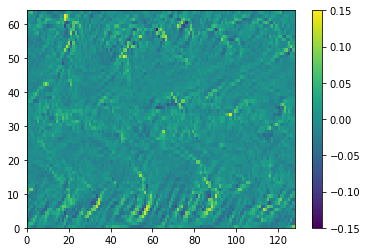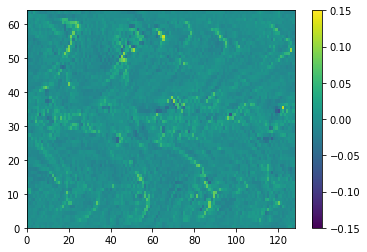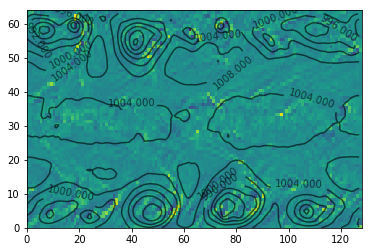In this notebook, I will develop tools to compute the exact advective tendencies using the velocities around the edge.

In :
from xnoah.sam import coarsen

In :
import matplotlib.pyplot as plt
%matplotlib inline


In :
import glob, os, re
from toolz import assoc, groupby, keymap, valmap
from toolz.curried import get, map, groupby
from collections import defaultdict
import xarray as xr

directory = "/home/disk/eos7/guest/SAM6.10.6_NG/continuation/OUT_3D"
files = os.listdir(directory)

# define a regex for reading the filenames
pattern = re.compile(r"(?P<run>.*)_(?P<time>\d+)_(?P<field>\w+)\.nc")

# parse files to list of dicts
file_matches = map(pattern.search, files)
file_info = [assoc(m.groupdict(), 'path', m.string) for m in file_matches]

# get list of runs
runs = groupby(get('run'), file_info)
runs = valmap(groupby('time'), runs)

time_steps = keymap(int, runs['NG_5120x2560x34_4km_10s_QOBS_EQX_1280'])
time_steps = valmap(map(lambda x: os.path.join(directory, x['path']), ), time_steps)
time_steps = valmap(list, time_steps)

In :
first_step = sorted(time_steps)
ds = xr.open_mfdataset(time_steps[first_step])
first_step

Out:
519480

In :
files_2d = glob.glob("/home/disk/eos13/guest/SAM6.10.6_NG/OUT_2D/*")
pattern = re.compile(r".*\/(?P<run>.*)_(?P<time>\d+)\.2Dcom.*nc")

# find the corresponding 2d files
for f in files_2d:
m = pattern.search(f)
if m:
if m.group('run') == 'NG_5120x2560x34_4km_10s_QOBS_EQX_1280':
if int(m.group('time')) == first_step:
a = m.string

data_2d = xr.open_dataset(a)
stat = xr.open_dataset("/home/disk/eos13/guest/SAM6.10.6_NG/OUT_STAT/NG_5120x2560x34_4km_10s_QOBS_EQX.nc")


# Break Data into blocks¶

In :
from xnoah.sam import coarsen
import numpy as np

U = ds.U.data
V = ds.V.data
W = ds.W.data

blocks = {2:40, 3: 40}
nt, nz, ny, nx = U.shape

def blocks_view(U):
return U.reshape((nt, nz, ny//blocks, blocks, nx//blocks, blocks))

U = blocks_view(U)
V = blocks_view(V)
W = blocks_view(W)

U_int_avg= U[:,:,:,:,:,0].mean(3)
V_int_avg= V[:,:,:,0,:,:].mean(-1)
W_avg = W.mean(-3).mean(-1)


Compute vertical grid values

In :
z = ds.z.values
z = np.hstack((0, z, z[-1]*2 - z[-2]))
zh = .5*(z[1:] + z[:-1])
dz = np.diff(zh)

rho = stat.RHO[-1].values
rho.shape = (-1, 1, 1)
dz.shape = (-1, 1, 1)


Compute divergence

In :
dx = dy = 4000
from scipy.ndimage import correlate1d

def diff_inplace(x, axis=-1):
return correlate1d(x, [-1, 1], axis=axis, mode='wrap')

# compute divergence
ux = da.ghost.map_overlap(U_int_avg, diff_inplace, {3: 1}, {3: 'periodic'}, axis=3)/dx/40
vy = da.ghost.map_overlap(V_int_avg, diff_inplace, {2: 1}, {2: 'reflect'}, axis=2)/dx/40

d = ux + vy

wz = da.diff(W_avg*rho, axis=1)/dz[:-1]/rho[:-1]
d_int = -da.cumsum(d*rho*dz, axis=1)


Here is a plot of $-\int_0^z (u_x + u_y) \rho dz'$ at a given height.

In :
plt.pcolormesh(d_int[0,10], vmin=-.15, vmax=.15)
plt.colorbar()

Out:
<matplotlib.colorbar.Colorbar at 0x7ff0686bbb00>Here is the plot of the average $\rho_0 w$ at the same height.

In :
plt.pcolormesh(rho*W_avg[0,10], vmin=-.15, vmax=.15)
plt.colorbar()

Out:
<matplotlib.colorbar.Colorbar at 0x7ff0685bc4e0>As we can see there is a big difference between the divergence estimated vertical velocity and the actual vertical velocity. This problem seems to be worse in the extra tropics than in the tropics.

In :
ps = coarsen(data_2d.PSFC, blocks=dict(x=40, y=40)).compute()

fig, ax = plt.subplots()

ax.pcolormesh(d_int[0,10], vmin=-.15, vmax=.15)
cs = ax.contour(ps, colors='k', alpha=.6)
ax.clabel(cs)

Out:
<a list of 14 text.Text objects>By overlating the surface pressure, we can see the errors are strongest over regions with low surface pressure. I took a look at the the source code for SAM and it seems like in each time loop, the following steps execute in order: